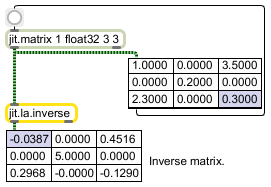# jit.la.inverse

Calculate the inverse of a matrix

## Description

The jit.la.inverse object calculates the inverse with respect to multiplication of a given input matrix. The input matrix must have typefloat32 or float64, and may have planecount 1 or 2. If the input matrix has a planecount of 2, it is assumed that the data is from the set of complex numbers.

## Matrix Operator

matrix inputs:1, matrix outputs:1
 Name IOProc Planelink Typelink Dimlink Plane Dim Type out n/a 1 1 1 1 1 float32 float64

## Attributes

Name Type g/s Description
thresh float The threshold value beneath which the absolute value of the result of internal calculations are considered to be equal to zero (default = 0.000000001)

## Examples